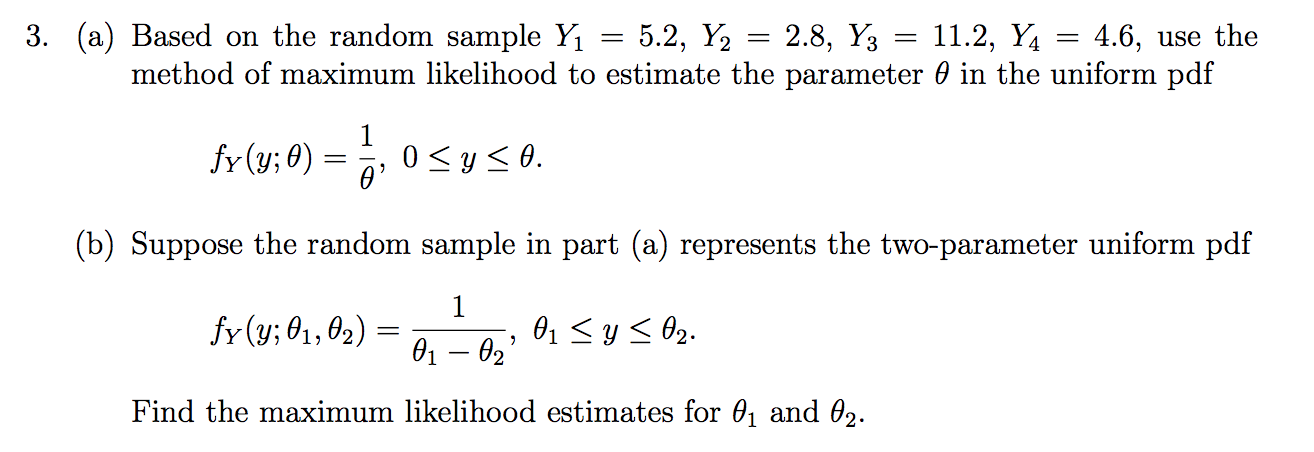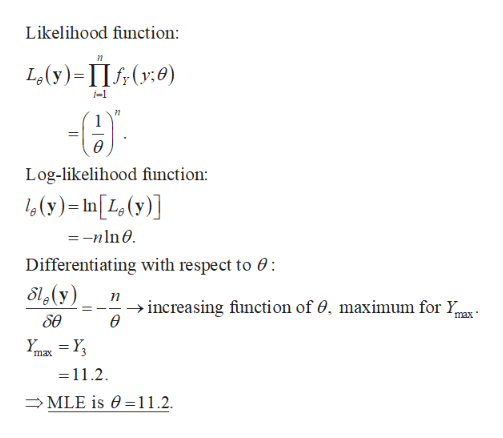# 3. (a) Based on the random sample Y1 = 5.2, Y24.6, use themethod of maximum likelihood to estimate the parameter 0 in the uniform pdf2.8, Y311.2, Yfr (y;0)0 ys0.(b) Suppose the random sample in part (a) represents the two-parameter uniform pdf1fr (y; 01, 02)01y02.01 02Find the maximum likelihood estimates for 01 and 02.

Questionhelp_outlineImage Transcriptionclose3. (a) Based on the random sample Y1 = 5.2, Y2 4.6, use the method of maximum likelihood to estimate the parameter 0 in the uniform pdf 2.8, Y3 11.2, Y fr (y;0) 0 ys0. (b) Suppose the random sample in part (a) represents the two-parameter uniform pdf 1 fr (y; 01, 02) 01y02. 01 02 Find the maximum likelihood estimates for 01 and 02. fullscreen
check_circleExpert Solution
Step 1

Note:

The pdf in Part (b) will be fY (y; θ1, θ2) = 1/(θ2 θ1) if θ1y θ. We have solved using this pdf.

Step 2

Introduction:

The maximum likelihood estimator (MLE) of a parameter θ is an estimator that maximizes the likelihood or probability of the set of observed data.

Step 3

Part (a):

The MLE for θ is Y3 = 11.2 calculated below:

...help_outlineImage TranscriptioncloseLikelihood function L(y)- II(y0) -1 Log-likelihood function: (y)-In[L(y)] -nln Differentiating with respect to 0 S(y) increasing fiunction of 0, maximum for Y ө max se max 11.2 MLE is e11.2. fullscreen

### Want to see the full answer?

See Solution

#### Want to see this answer and more?

Solutions are written by subject experts who are available 24/7. Questions are typically answered within 1 hour*

See Solution
*Response times may vary by subject and question
Tagged in

### Hypothesis Testing